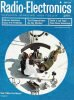Please Support RF Cafe by purchasing my  ridiculously low−priced products, all of which I created.

RF Workbench  (shareware)May 1964 Radio-Electronics[Table of Contents] Wax nostalgic about and learn from the history of early electronics. See articles from Radio-Electronics, published 1930-1988. All copyrights hereby acknowledged.

All three questions in the May 1964 Radio−Electronics magazine "What's Your EQ?" challenge should not be too difficult for most RF Cafe visitors. EQ, by the way, stands for "Electronics Quotient." For the first one, you might want to make use of the Delta-Wye converter in the newly released (and free) Espresso Engineering Workbook™. With the Voltage Divider, don't bother trying to write multiple equations in multiple unknowns. By inspection you can deduce what the voltage drop across the 2.5 kΩ resistor are, then knowing that the current through all resistors is the same, figure out the required values of R1 and R3. Finally, sum all the resistances and multiply by the current. Done. I have to admit to not getting the Doodle answer. If I had time, I'd do the oscilloscope thing to verify the author's solution.Three puzzlers for the student, theoretician and practical mono Simple? Double-check your answers before you say you've solved them. If you have an interesting or unusual puzzle (with an answer) send it to us. We will pay \$10 for each one accepted. We're especially interested in service stinkers or engineering stumpers on actual electronic equipment. We get so many letters we can't answer individual ones, but we'll print the more interesting solutions - ones the original authors never thought of.

Write EQ Editor, Radio-Electronics, 154 West 14th Street, New York, N. Y. 10011

Fig. 1 shows an unterminated tee network and Fig. 2 shows an unterminated pi network. Can you determine the electrical characteristic that the two networks have in common? - K. Collins

In the diagram (to the left), R2 and R4 are each 2,500 ohms. Readings taken with voltmeters (V) assumed to have infinite impedance show 70 volts across R1 plus R2, and 30 volts across R2 plus R3. What is the input voltage (EIN)? - Harold J. Turner, Jr.

How do you produce this waveform on an oscilloscope? - Lee H. Stanton

Quizzes from vintage electronics magazines such as Popular Electronics, Electronics-World, QST, and Radio News were published over the years - some really simple and others not so simple. Robert P. Balin created most of the quizzes for Popular Electronics. This is a listing of all I have posted thus far.

Each network has a characteristic impedance of 600 ohms. This can be determined by connecting a 600-ohm load resistor across the output terminals of each and measuring the effective resistance between the input terminals of each network. Both networks will have an input resistance of 600 ohms. Since this is the same value as the load resistance, the characteristic impedance of each network is said to be 600 ohms.

In the tee network, the 600-ohm load resistor is in series with the 200 ohm resistor on the right-hand side of the network, making the equivalent of 800 ohms. This resistance is in combination with the 800-ohm shunt resistor, making a series-shunt combination that equals 400 ohms. By adding the left-hand resistance of 200 ohms to 400 ohms, the resistance between the input terminals is found to be 600 ohms. Since this is the same value as the load resistor, the characteristic impedance of the tee network is 600 ohms.

In the pi network, a 600-ohm load resistor is in parallel with the right-hand 1,800-ohm shunt resistor. This combination equals 450 ohms and is in series with the 450-ohm network resistor, making a total of 900 ohms. The left-hand shunt resistance of 1,800 ohms is now in parallel with 900 ohms, which makes the input resistance across the input terminals 600 ohms. Since the load resistance of 600 ohms equals the input resistance, the characteristic impedance of the pi network is 600 ohms.

Note:

By using the Wye-Delta or Delta-Wye transformation, it can also be shown that the two networks are equivalent. The tee and pi networks are examples of Wye and Delta networks respectively. - Editor

This puzzle is not as hard as it seems at first glance. Since the four resistors are connected in series, the same current must flow through each. We see that R4 has the same resistance as R2. Therefore the drop across R3 plus R4 is the same as the drop across R2 plus R3 - 30 volts. The total input voltage is 100.

If the horizontal input of your scope is not disconnected from the horizontal amplifier when using internal sweep (about 10 or 12 cycles), simply connect the 60-cycle test signal to the vertical input, and also to the horizontal input through a small capacitor for a 90° phase shift.

If the horizontal input is disconnected when using internal sweep, connect the capacitor from the 60-cycle test to the horizontal amplifier internally with a clip lead.

Posted July 7, 2023